## LaTeX入门

$Update\ 2019/6/29:$ 发现 $\mathtt{TeX\ Live}$ 更新了 $2019$ 版本，因此原先的下载链接都失效了 $Q\omega Q$ ，所以更新了下链接并重新上传了云盘。因为换代所以安装界面也变了，因此我也顺便更新了下安装教程。
$Update\ 2019/9/08:$ 最近博客园不稳定，而我这儿的图片全都是存在博客园上的，于是全都挂了 $Q\omega Q$ 。现在已更换了图床，但加载速度可能比较慢。

# 前言

$\qquad$ 作为一名 $\mathtt{OIer}$ ，你肯定对 $\mathtt{LaTeX}$ 不陌生，在写题面或是题解时，使用该语言可以生成很美观的数学公式，大大提高了阅读体验（说实话现在我看到题目题解没有用 $\mathtt{LaTeX}$ 写数学公式就头大）。
$\qquad$ 不过，作为一门能生成媲美书籍的质量的文章的语言， $\mathtt{LaTeX}$ 的魅力可不仅仅局限于它的数学公式。

# 声明

$\qquad$ 实际上我只是 $\mathtt{LaTeX}$ 入门了一段时间，用其写过几篇文章，查阅过较多资料而已。我绝不是什么精通 $\mathtt{LaTeX}$ 的人，我也绝不保证这篇文章所述的都是确切的，而我也希望尽量避免对大家造成误导，尽量多查阅资料并选用合适的词汇来叙述。因此希望大家在看这篇文章时多查阅相关资料，综合起来理解，而非盲目相信。如有错误请在评论区指出，谢谢。

# $\mathbf{LaTeX}$ 简介

$\qquad$ $\mathtt{TeX}$ 是一个由美国计算机教授高德纳编写的功能强大的排版软件，而为了让使用者可以更为方便的使用 $\mathtt{TeX}$ 的强大功能，美国计算机科学家莱斯利·兰伯特在20世纪80年代初期开发了一种基于 $\mathtt{TeX}$ 的排版系统—— $\mathtt{LaTeX}$ 。
$\qquad$ $\mathtt{LaTeX}$ 是当今世界上最流行和使用最为广泛的 $\mathtt{TeX}$ 宏集。使用 $\mathtt{LaTeX}$ 基本上不需要使用者自己设计命令和宏等，因为 $\mathtt{LaTeX}$ 已经替你做好了。因此，即使使用者并不是很了解 $\mathtt{TeX}$ ，也可以在短短的时间内生成高质量的文档。而对于生成复杂的数学公式， $\mathtt{LaTeX}$ 表现的更为出色。
$\qquad$ $\mathtt{LaTeX}$ 编辑和排版的核心思想在于通过章、节等简单的概念指定文档的逻辑结构，规定了每一句话在文章中所从属的层次，从而极大方便了对各个层次批量处理，而这些结构的格式和布局，则是由 $\mathtt{LaTeX}$ 系统负责处理。因此，它鼓励从内容中分离布局，同时仍然允许在需要时进行手动排版调整。
$\qquad$ 其实 $\mathtt{LaTeX}$ 的这种工作方式类似与网页，均是源文件经由引擎渲染产生最终效果。而 $\mathtt{LaTeX}$ 的语法规则其实和 $\mathtt{HTML}$ 比较像。因此与 $\mathtt{HTML}$ 一样， $\mathtt{LaTeX}$ 的入门其实很简单。
$\qquad$ 而关于 $\mathtt{LaTeX}$ 的写法，实际上它最准确的写法应该是 $\LaTeX$ （且洛谷这里莫名后面有空白），但个人认为这样写阅读体验并不算太好，所以个人还是会习惯写为 $\mathtt{LaTeX}$ （若因技术限制而无法做到，也是可以这么写的），但注意不要改变任何一个字母的大小写，以免与单词 $\mathtt{latex}$ 混淆。

$\qquad$ 以上内容取自 $\mathtt{Wiki}$ 、百度百科和一些博客，加以自己的看法整理而成。
$\qquad$ 若想要知道更多关于 $\mathtt{LaTeX}$ 的介绍，请自行查阅 $\mathtt{Wiki}$ ，或是 $\mathtt{Google}$ /百度。

# $\mathbf{LaTeX}$ 软件的安装和使用

## 选择一个 $\mathbf{TeX}$ 发行版

$\qquad$ 在 $\mathtt{Windows}$ 下常用 $\mathtt{CTeX}$ 或 $\mathtt{TeX\ Live}$ ，在 $\mathtt{Linux}$ 下常用 $\mathtt{TeX\ Live}$ 。
$\qquad$ 因为我使用的是 $\mathtt{Windows}$ ，且对 $\mathtt{Linux}$ 一窍不通，所以这里只讲 $\mathtt{Windows}$ 下的安装。
$\qquad$ 对于 $\mathtt{CTeX}$ 和 $\mathtt{TeX\ Live}$ ，因为 $\mathtt{CTeX}$ 已经很久很久没有更新了（官网上的更新记录停在了 $2012$ 年），所以建议使用 $\mathtt{TeX\ Live}$ 套装。

## 安装 $\mathbf{TeX\ Live}$

$\qquad$ $\mathtt{TeX\ Live}$ 官网传送门
$\qquad$ 可以直接从官网下载安装程序或是镜像文件，这里我使用的是镜像文件。
$\qquad$ $\mathtt{TeX\ Live2019}$ 镜像文件清华镜像站下载
$\qquad$ 注意这个链接直接链向下载。
$\qquad$ 另外，我也把镜像文件上传到百度云盘了。
$\qquad$ $\mathtt{TeX\ Live2019}$ 镜像文件百度云盘下载 $\quad$ 提取码: 1rbd。

$\qquad$ 下载完成后就可以安装了。
$\qquad$ 装载镜像文件，以管理员身份运行 $\mathtt{install-tl-advanced.bat}$ ，等待它加载（会弹出 $\mathtt{cmd}$ 窗口），然后就会有这样一个窗口：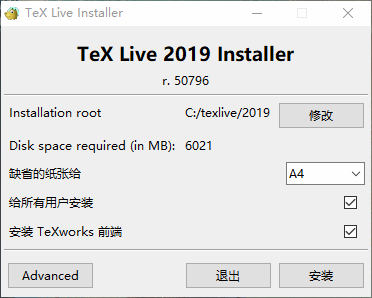$\qquad$ 按自己喜好自定义安装目录（我觉这个修改做的很傻。。），如果你是打算使用我在之后说的编辑器，那么可以取消“安装 $\mathtt{TeXworks}$ 前端”这一选项。然后点击安装即可。
$\qquad$ 安装通常要比较长的时间，请耐心等待。
$\qquad$ 安装完成后，可以打开 $\mathtt{cmd}$ ，输入命令tex -version，若返回的是 $\mathtt{TeX}$ 的版本信息（如图），那么代表安装成功。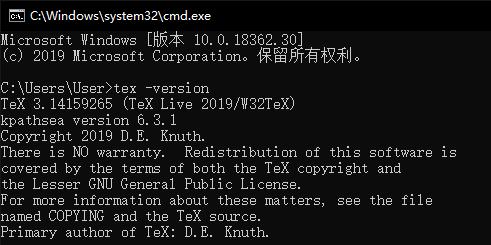## 选择一个 $\mathbf{LaTeX}$ 编辑器

$\qquad$ 在安装好 $\mathtt{LaTeX}$ 环境后，通常其自带一个编辑器，如 $\mathtt{CTeX}$ 的 $\mathtt{WinEdt}$ ， $\mathtt{TeX\ Live}$ 的 $\mathtt{TeXworks}$ 。
$\qquad$ 但我们安装的 $\mathtt{TeX\ Live}$ 自带的 $\mathtt{TeXworks}$ 在什么都没配置的情况下只有最基本的功能，且配置也是挺麻烦的（这个编辑器的特色好像就是脚本），因此这里我推荐 $\mathtt{TeXstudio}$ （这个还是跨平台的）。
$\qquad$ 至于我为什么推荐 $\mathtt{TeXstudio}$ ，可以自行去知乎上搜搜，这个编辑器的功能还是很强大的，颜值虽然不算高，但好歹能看。
$\qquad$ 另外 $\mathtt{VSC}$ 、 $\mathtt{Sublime}$ 等编辑器在配置好后也是可以编辑 $\mathtt{LaTeX}$ 的。

## 安装 $\mathbf{TeXstudio}$

$\qquad$ $\mathtt{TeXstudio}$ 官网传送门
$\qquad$ 官网下载入口应该很显眼，就不弄链接了。
$\qquad$ 我同样将其安装包传到了百度云盘。
$\qquad$ $\mathtt{TeXstudio}$ 安装包百度云盘下载 $\quad$ 提取码: iyqm。

$\qquad$ 下载完成后就可以安装了。
$\qquad$ 打开安装包，自定义安装目录，然后安装就好（这个安装就快的多了）。

## 设置 $\mathbf{TeXstudio}$

$\qquad$ 打开 $\mathtt{TeXstudio}$ ，会发现是英文的，那么我们先将其修改为中文：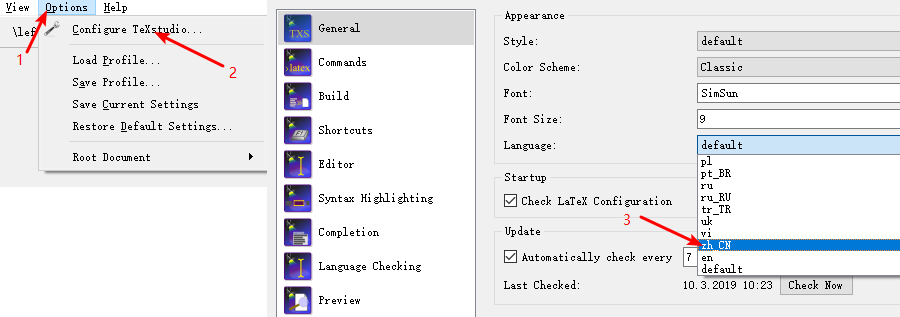$\qquad$ 通常 $\mathtt{TeXstudio}$ 会自动链上先前安装的 $\mathtt{TeX\ Live}$ ，因此编译命令啥的就不用修改了。
$\qquad$ $\mathtt{TeXstudio}$ 的默认编译器是 $\mathtt{PdfLaTeX}$ ，但建议使用 $\mathtt{xeLaTeX}$ ，因为其对中文的支持更为成熟，可以按下图修改。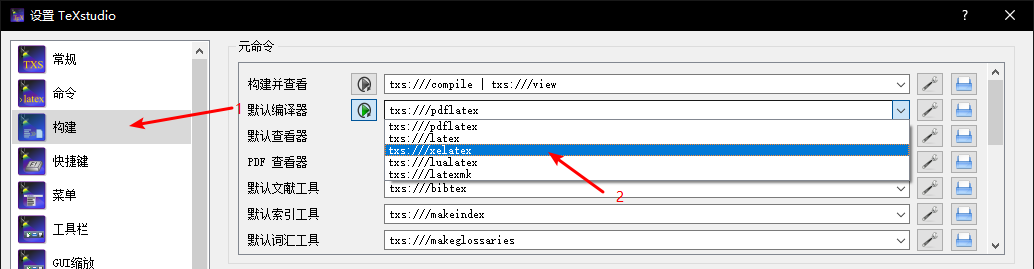$\qquad$ 而 $\mathtt{TeXstudio}$ 的默认 $\mathtt{pdf}$ 查看器是其自带的，若是想用外部的，则在刚刚修改默认编译器的页面将原本的 $\mathtt{pdf}$ 查看器 $\mathtt{txs:///view-pdf-internal --embedded}$ 修改为 $\mathtt{txs:///view-pdf-external}$ 即可。
$\qquad$ 另外，外部的 $\mathtt{pdf}$ 查看器一般它是设置为你电脑上的默认 $\mathtt{pdf}$ 查看器（可以在设置-命令-外部 $\mathtt{PDF}$ 查看器 $\$ 查看），若是想要更改，就把原本命令中目录的那一部分替换为你想要使用的 $\mathtt{pdf}$ 查看器所在的目录即可。
$\qquad$ 至于字体、高亮、快捷键啥的设置这里就不多说了（另外设置左下角可以开启高级设置），可以自己研究或网上查。

# $\mathbf{LaTeX}$ 基本使用

## 第一个 $\mathbf{pdf}$

$\qquad$ 打开 $\mathtt{TeXstudio}$ ，直接按快捷键 $\mathtt{Ctrl+N}$ 新建一个文档。然后将下列内容复制进去。

\documentclass{article}
\begin{document}
Hello, World!
\end{document}

$\qquad$ 然后直接按快捷键 $\mathtt{Ctrl+S}$ 保存文档。
$\qquad$ 保存文件类型选择 $\mathtt{tex}$ 文件，建议专门建一个文件夹放里面，等会就知道为什么了。
$\qquad$ 在写保存文件名是需要注意尽量避免中文、特殊字符，建议只写英文，不然的话在编译该文件时可能会编译失败，或是生成的 $\mathtt{pdf}$ 有乱码。
$\qquad$ 然后就可以编译了。 $\mathtt{TeXstudio}$ 的选项有三种：
$\qquad\qquad$ 1. 构建并查看（快捷键 $\mathtt{F5}$ ），可以类比于 $\mathtt{Dev\ C++}$ 里的 $\mathtt{F11}$ （编译并运行）。
$\qquad\qquad$ 2. 编译（快捷键 $\mathtt{F6}$ ），可以类比于 $\mathtt{Dev\ C++}$ 里的 $\mathtt{F9}$ （编译）。
$\qquad\qquad$ 3. 查看（快捷键 $\mathtt{F7}$ ），可以类比于 $\mathtt{Dev\ C++}$ 里的 $\mathtt{F10}$ （运行）。
$\qquad$ 我们直接选择构建并查看，然后 $\mathtt{TeXstudio}$ 就会开始编译并在编译完成后直接使用你设置好的阅读器打开生成的 $\mathtt{pdf}$ 。
$\qquad$ 如果顺利的话，你就成功用 $\mathtt{LaTeX}$ 生成了你的第一个 $\mathtt{pdf}$ ！
$\qquad$ 效果预览（洛谷的编辑器不支持 $\mathtt{HTML}$ ，不能把图片放中间真的超难看）：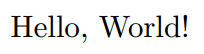$\qquad$ 下面简单介绍这几个命令：
$\qquad$ $\mathbf{\backslash documentclass\{\}}$ ，指定文档类型（模板），在之后会有介绍，这里暂不展开。
$\qquad$ $\mathbf{\backslash begin\{document\}\ \backslash end\{document\}}$ ，在这两个命令之间就是你的文档内容，而它前面的部分称为导言区
$\qquad$ 另外，记得在编译源文件前把之前运行而打开的 $\mathtt{pdf}$ 关闭。

## 标题、作者、日期

$\qquad$ 建立一个新文档或是直接在之前建立的文档里将下列内容复制进去。

\documentclass{article}
\author{My Name}
\title{TEST}
\begin{document}
\maketitle
Hello, World!
\end{document}

$\qquad$ 编译并查看 $\mathtt{pdf}$ 。
$\qquad$ 效果预览：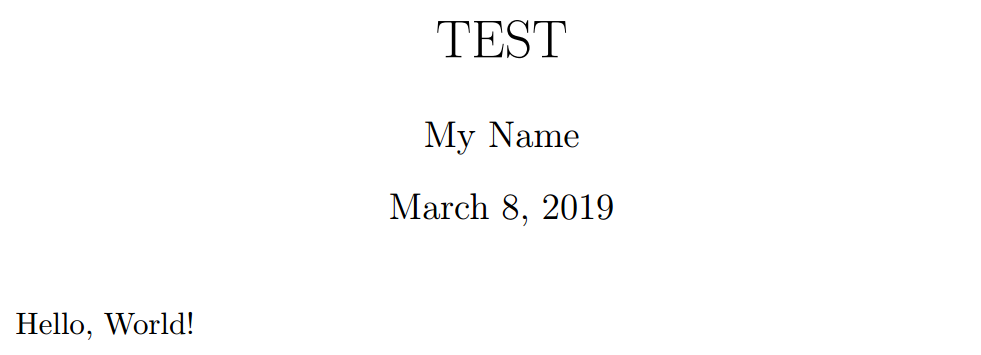$\qquad$ 下面简单介绍这几个命令：
$\qquad$ $\mathbf{\backslash auther\{\}}$ ，指定作者姓名。
$\qquad$ $\mathbf{\backslash title\{\}}$ ，指定文章标题。
$\qquad$ $\mathbf{\backslash maketitle\{\}}$ ，排版标题和、作者、日期。
$\qquad$ 一般情况下，日期会自动选取你编辑这篇文档的当天并直接排版上去，若是想要自己设置，可以在 $\mathbf{\backslash title\{\}}$ 的下面加上命令 $\mathbf{\backslash date\{\}}$ ，以指定日期。

## 章节、段落

$\qquad$ 建立一个新文档或是直接在之前建立的文档里将下列内容复制进去。

\documentclass{article}
\author{My Name}
\title{TEST}
\begin{document}
\maketitle
\section{First}
Hello, World!
\subsection{Second}
gugugugugugu
\subsubsection{Third}
\paragraph{HI}
Hi!
\subparagraph{AH}
Ah!
\end{document}

$\qquad$ 编译并查看 $\mathtt{pdf}$ 。
$\qquad$ 效果预览：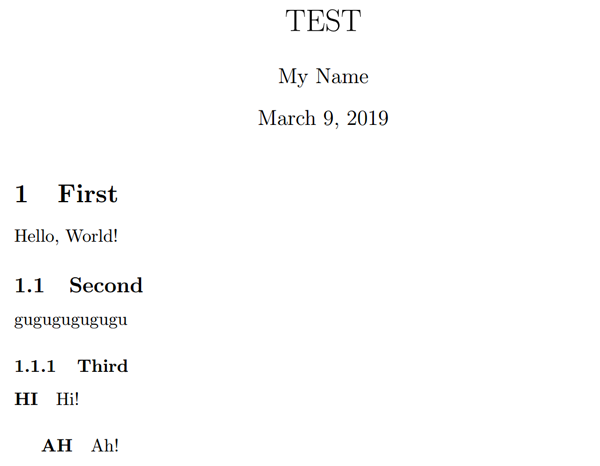$\qquad$ 下面简单介绍这几个命令：
$\qquad$ $\mathbf{\backslash section\{\}}$ ，一级章节标题。
$\qquad$ $\mathbf{\backslash subsection\{\}}$ ，二级章节标题。
$\qquad$ $\mathbf{\backslash subsubsection\{\}}$ ，三级章节标题。
$\qquad$ $\mathbf{\backslash paragraph\{\}}$ ，一级段落。
$\qquad$ $\mathbf{\backslash subparagraph\{\}}$ ，二级段落。
$\qquad$ 若是想要去除标题前面的数字编号，则是在原命令中大括号前加一个'*'即可，例如 $\mathbf{\backslash section}$ * $\mathbf{\{\}}$ 。
$\qquad$ 但注意的是，去除编号后章节标题的默认是不会加到目录中的。

## 目录

$\qquad$ 建立一个新文档或是直接在之前建立的文档里将下列内容复制进去。

\documentclass{article}
\author{My Name}
\title{TEST}
\begin{document}
\maketitle
\tableofcontents
\section{First}
Hello, World!
\subsection{Second}
gugugugugugu
\subsubsection{Third}
\paragraph{HI}
Hi!
\subparagraph{AH}
Ah!
\end{document}

$\qquad$ 编译并查看 $\mathtt{pdf}$ 。
$\qquad$ 效果预览：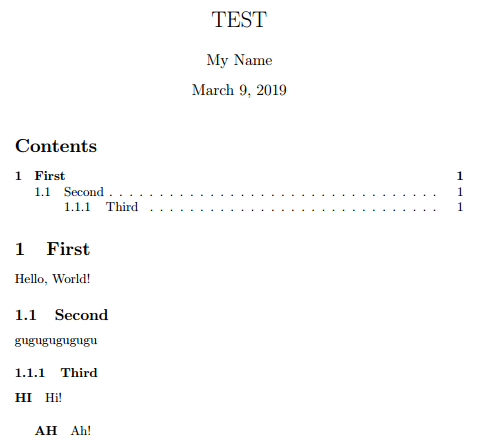$\qquad$ 下面简单介绍这个命令：
$\qquad$ $\mathbf{\backslash tableofcontents\{\}}$ ，根据你的章节信息自动生成目录。
$\qquad$ 需要注意的是， $\mathtt{LaTeX}$ 处理目录需要编译两次
$\qquad$ 关于目录在后面还会有一些讲解。

## 换行、注释

$\qquad$ 建立一个新文档或是直接在之前建立的文档里将下列内容复制进去。

\documentclass{article}
\begin{document}
gugugugu
gugugu

233333    %这里是注释

gugu\\
gugu 2333     23333\par
gugugugugu
\end{document}

$\qquad$ 编译并查看 $\mathtt{pdf}$ 。
$\qquad$ 效果预览：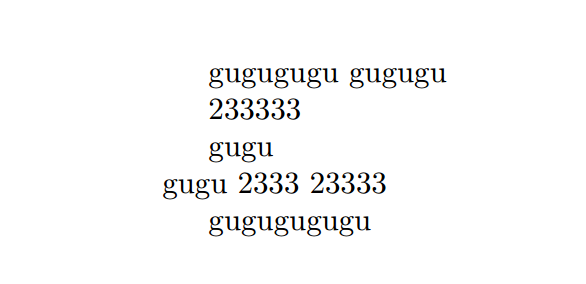$\qquad$ 下面简单介绍 $\mathtt{LaTeX}$ 的换行和注释：
$\qquad$ 若是在源文件中直接换行，那么 $\mathtt{LaTeX}$ 会将这两行文字之间的空白字符忽略，然后以一个空格隔开接在一行。
$\qquad$ 若是在源文件中两行文字之间空一行，那么第二行文字会被认为是新起的一个段落，会带有缩进
$\qquad$ 命令 $\backslash\backslash$ 是常用的换行命令，但不是新起一段，换行后的那段文字没有缩进，在 $\mathtt{TeXstudio}$ 中有快捷键 $\mathtt{Ctrl+Enter}$ 。
$\qquad$ 命令 $\mathbf{\backslash par}$ 也是换行命令，准确的说，该命令的意思是新起一个段落（和空行的效果一样），因此会带有缩进
$\qquad$ 在 $\mathtt{LaTeX}$ 中，若源文件中文字之间有若干个空格，会被直接认为只有一个空格。
$\qquad$ 注释则以 $\%$ 号开头，在该符号后（仅一行）的内容均会被认为是注释。

## 缩进

$\qquad$ 建立一个新文档或是直接在之前建立的文档里将下列内容复制进去。

\documentclass{article}
\begin{document}
gugugugu\\
\indent gugugu\par
\noindent gugugugu\\
\indent\indent\indent gugugu
\end{document}

$\qquad$ 编译并查看 $\mathtt{pdf}$ 。
$\qquad$ 效果预览：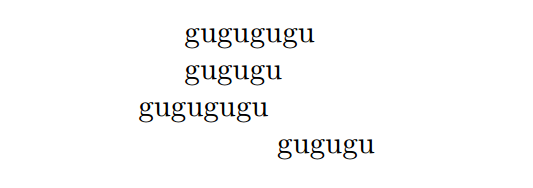$\qquad$ 下面简单介绍这几个命令：
$\qquad$ $\mathbf{\backslash indent\{\}}$ ，插入一个缩进，其实可以把该命令看作是一个 $\mathtt{tab}$ 键。
$\qquad$ $\mathbf{\backslash noindent\{\}}$ ，取消缩进。

## 数学公式

$\qquad$ 基本与在 $\mathtt{markdown}$ 里插入 $\mathtt{LaTeX}$ 的数学公式的语法相同，这里就不详细阐述。
$\qquad$ 另外，我曾写过一个针对洛谷的 $\mathtt{LaTeX}$ 数学公式大全，不过对于在 $\mathtt{LaTeX}$ 里的运用基本也够，这里就挂个链接吧，希望能对大家有所帮助。
$\qquad$ $\mathtt{LaTeX}$ 数学公式大全

## 图片

$\qquad$ 建立一个新文档或是直接在之前建立的文档里将下列内容复制进去。

\documentclass{article}
\usepackage{graphicx}
\begin{document}
First\\\\
\includegraphics[width=4cm,height=2cm]{1.png}\\
\indent Second\\\\
\includegraphics[width=4cm,height=3cm]{2.png}
\end{document}

$\qquad$ 编译并查看 $\mathtt{pdf}$ 。
$\qquad$ 效果预览：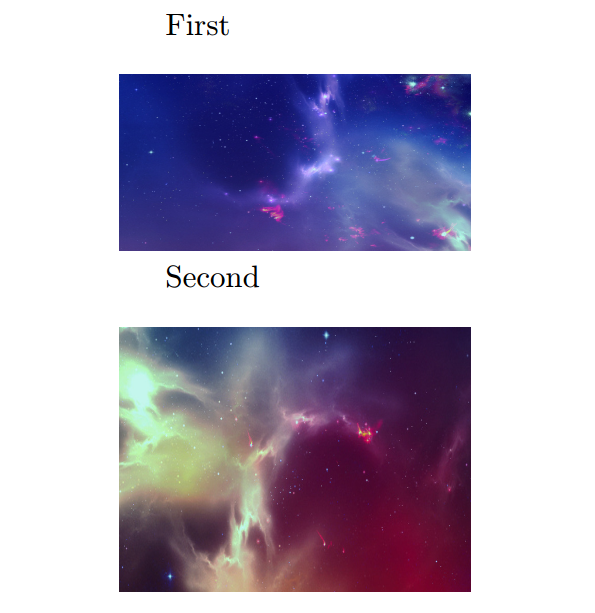$\qquad$ 下面简单介绍这几个命令：
$\qquad$ $\mathbf{\backslash usepackage\{\}}$ ，见下文。
$\qquad$ $\mathbf{\backslash includegraphics[\ ]\{\}}$ ，插入图片， $[\ ]$ 里设置图片的参数， $\{\}$ 里则时图片的路径，以 $\mathtt{tex}$ 文件保存的位置开始

## 表格

$\qquad$ 建立一个新文档或是直接在之前建立的文档里将下列内容复制进去。

\documentclass{article}
\begin{document}
\begin{tabular}{|c|c|}
a & b \\
c & d\\
\end{tabular}\\
\indent \begin{tabular}{|c|c|}
\hline
a & b \\
\hline
c & d\\
\hline
\end{tabular}\\
\begin{center}
\begin{tabular}{|c|c|}
\hline
a & b \\
\hline
c & d\\
\hline
\end{tabular}
\end{center}
\end{document}

$\qquad$ 编译并查看 $\mathtt{pdf}$ 。
$\qquad$ 效果预览：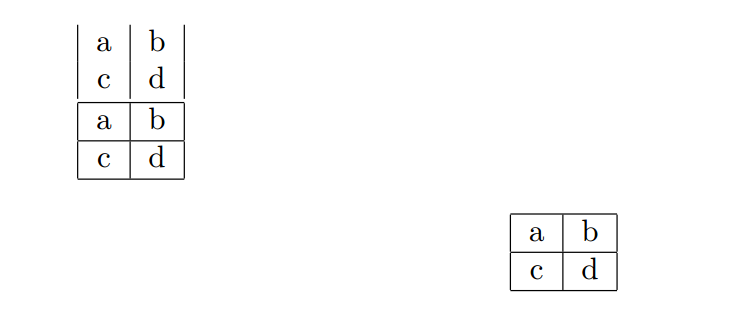$\qquad$ 下面简单介绍这几个命令：
$\qquad$ 表格通常声明在 $\mathbf{tabular}$ 环境（ $\mathtt{begin\ end}$ 命令是为创建一个某某环境）中，而 $\mathtt{|c|c|}$ 这些东西是表格的参数， $\mathtt{c}$ 表示一列，且表示该列的单元的内容是居中的，若填写的是 $\mathtt{l}$ ，则表示左对齐，若是 $\mathtt{r}$ ，则表示右对齐； $|\$ 则是画一条竖线。
$\qquad$ 表格内容以 $\&$ 来分隔每一列的内容， $\backslash\backslash$ 分隔行， $\mathbf{\backslash hline}$ 命令是画一条水平线。
$\qquad$ $\mathbf{center}$ ，居中环境，在后文会有介绍。
$\qquad$ 当然对表格的操作绝不仅限于此，在之后也会有介绍。

## 中文支持

$\qquad$ 如果细心的话，你会发现我上面给的所有例子都是英文的，因为 $\mathtt{article}$ 模板并不支持中文。
$\qquad$ 如果是以前的 $\mathtt{LaTeX}$ ，要让它支持中文是一件挺麻烦的事情，但现在就好办了，只需将开头的 $\mathbf{\backslash documentclass\{article\}}$ 换成 $\mathbf{\backslash documentclass[UTF8]\{ctexart\}}$ 就可以了（方括号是让其使用 $\mathtt{UTF8}$ 编码，虽然好像不加也没事，但我还是习惯会加）。
$\qquad$ 建立一个新文档或是直接在之前建立的文档里将下列内容复制进去。

\documentclass[UTF8]{ctexart}
\author{我}
\title{测试}
\begin{document}
\maketitle
你好，世界！
\end{document}

$\qquad$ 编译并查看 $\mathtt{pdf}$ 。
$\qquad$ 效果预览：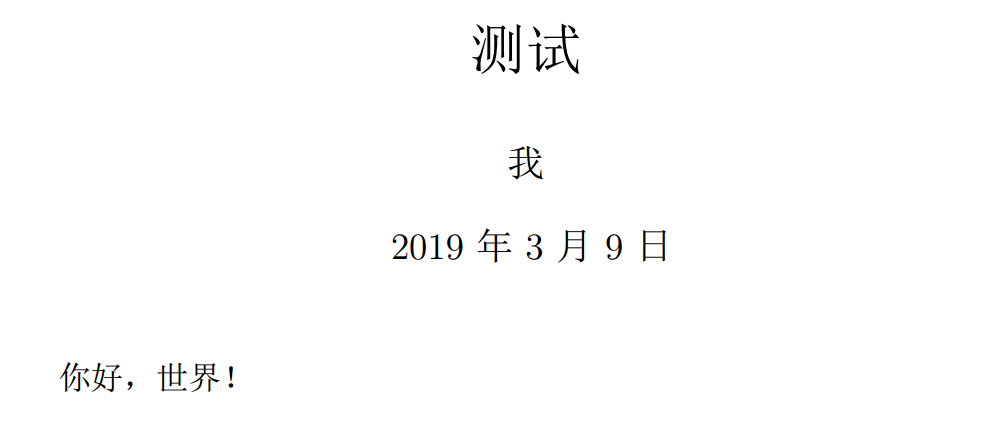$\qquad$ 然后你就可以中英文混排了。

## 宏包

$\qquad$ 之前在图片那一栏出现的 $\mathbf{\backslash usepackage\{\}}$ 就是调用宏包的命令。
$\qquad$ 宏包其实就可以理解为 $\mathtt{C++}$ 的库，里面定义了一些命令（好比于 $\mathtt{C++}$ 的库函数）来达到一定的功能，通常在声明模板（即命令 $\mathbf{\backslash documentclass\{\}}$ ）后，正文前就将所有要用的宏包调用（说实话和 $\mathtt{C++}$ 调用库很像）。
$\qquad$ 对于编辑数学公式比较常用的宏包有： $\mathtt{amsmath}$ 和 $\mathtt{amssymb}$ 。
$\qquad$ 因为另外一些常用宏包的功能我会在后面介绍，因此这里就不罗列了。

## 模板

$\qquad$ $\mathbf{\backslash documentclass\{\}}$ 命令就是声明该文档所用的模板，在之前的教程里我们就使用了两个模板， $\mathtt{article}$ 和 $\mathtt{ctexart}$ 。
$\qquad$ 模板通常可以规定章节一级标题都用什么字体什么字号怎么对齐，章节二级标题用什么字体什么字号怎么对齐，一级段落又用什么字体什么字号怎么对齐，页眉页脚页边距一类的页面设置又怎么设置，以及一些自定义的命令。
$\qquad$ 介于大多数情况我们只需使用 $\mathtt{ctexart}$ 模板，这里就不多阐述各种模板了。
$\qquad$ 另外，该命令也提供了一个文档格式的设置，即 $\mathbf{\backslash documentclass[options]\{\}}$ （其实在 $\mathtt{LaTeX}$ 里的很多命令都是如此，方括号里可以填写设置，不填可以省略方括号），在方括号里可以填写关于文档格式的设置，比较常见的是设置正文字号和页面规格，比如 $\mathbf{\backslash documentclass[12pt, a4paper]\{article\}}$ 表示正文字号为 $\mathtt{12pt}$ ，页面规格为 $\mathtt{A4}$ 纸。另外， $\mathtt{LaTeX}$ 默认页面大小是 $\mathtt{A4}$ 纸。

# 文本间的距离

## 缩进

$\qquad$ 前文有讲。

## 水平距离

$\qquad$ $\mathbf{\backslash hspace\{length\}}$ ，该命令会产生一个长度为 $\mathtt{length}$ 的水平间距以隔开前后文字。
$\qquad$ 示例代码：

\documentclass[UTF8]{ctexart}
\author{我}
\title{测试}
\begin{document}
\maketitle
\noindent 控制\hspace{5cm}水平距离。\\
\end{document}

$\qquad$ 效果预览：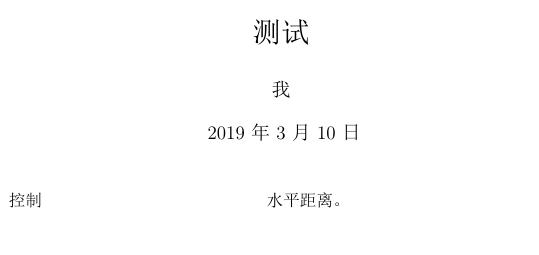$\qquad$ 另外，可以使用数学公式里的空格来控制水平间距。
$\qquad$ 其实在 $\mathtt{LaTeX}$ 里有挺多命令是可以插入空格的，但我认为并不需要知晓全部，只要知道几个常用的就好，所以就不多讲了。

## 垂直距离

$\qquad$ $\mathbf{\backslash vspace\{length\}}$ ，该命令用于两段文字间，产生一个长度为 $\mathtt{length}$ 的垂直间距以隔开前后两端，具体可见示例代码。

$\qquad$ 示例代码：

\documentclass[UTF8]{ctexart}
\author{我}
\title{测试}
\begin{document}
\maketitle
\noindent 若在同一段文字插入
\vspace{1cm}
则只会显示一个空格，并将效果继承到这一段。\\
和下一段之间。\\
\noindent 用空行来表示另起一段
\vspace{1cm}

\noindent 或者使用 $\backslash$ par来另起一段\par
\vspace{1cm}
\noindent 才会有效果。
\end{document}

$\qquad$ 效果预览：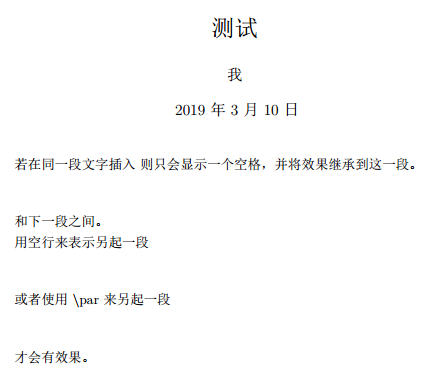## 换页

$\qquad$ $\mathbf{\backslash newpage}$ ，该命令会使当前页直接结束，并起新的一页。
$\qquad$ 示例代码：

\documentclass[UTF8]{ctexart}
\author{我}
\title{测试}
\begin{document}
\maketitle
直接新起
\newpage
一页。
\end{document}

$\qquad$ 效果预览：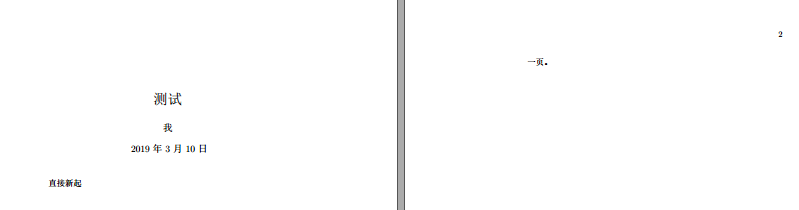# 页眉和页脚

## 页码

$\qquad$ $\mathbf{\backslash pagenumbering\{numstyle\}}$ ，该命令可以设置当前页面的页码显示为某一种 $\mathtt{numstyle}$ 。
$\qquad$ 共有 $5$ 种 $\mathtt{numstyle}$ ：
$\qquad\qquad$ 1. $\mathtt{arabic}$ ，阿拉伯数字，即 $1,2,3,4,\dots$ ，是默认样式。
$\qquad\qquad$ 2. $\mathtt{roman}$ ，小写罗马数字，即 $\mathrm{i,ii,iii,iv,\dots}$ 。
$\qquad\qquad$ 3. $\mathtt{Roman}$ ，大写罗马数字，即 $\mathrm{I,II,III,IV,\dots}$ 。
$\qquad\qquad$ 4. $\mathtt{alph}$ ，小写英文字母，即 $\mathrm{a,b,c,d,\dots}$ 。
$\qquad\qquad$ 5. $\mathtt{Alph}$ ，大写英文字母，即 $\mathrm{A,B,C,D,\dots}$ 。

$\qquad$ $\mathbf{\backslash setcounter\{page\}\{number\}}$ ，该命令可以设置当前页面的页码为哪个数字。
$\qquad$ 不过当该页页码在页眉时，这个命令要写在设置页码显示样式的命令前；若在页脚，则要写在设置页码显示样式的命令后（可能是这样的，至少我在使用的时候是遇到挺多问题的）。
$\qquad$ 示例代码：

\documentclass[UTF8]{ctexart}
\begin{document}
使用大写英文字母。
\pagenumbering{Alph}
\newpage
设置页码数为 $4$ ，并为大写罗马数字。
gugu %这个忘记删去了。。但懒得改图片，所以就酱紫吧
\pagenumbering{Roman}
\setcounter{page}{4}
\end{document}

$\qquad$ 效果预览：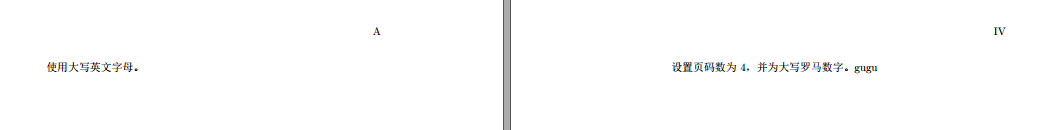$\qquad$ 另外， $\mathbf{\backslash setcounter}$ 这个命令实际上是 $\mathtt{LaTeX}$ 的计数器的一个操作，介于计数器好像不太常用（至少我在别的博客挺少看到的），这里就不展开了，有兴趣的同学可以去参考这篇博客： $\mathtt{LaTeX}$ 的计数器

## 页眉和页脚的简单样式

$\qquad$ $\mathbf{\backslash pagestyle\{option\}}$ 和 $\mathbf{\backslash thispagestyle\{option\}}$ ，该命令可以设置页面样式，即页眉和页脚，使用的是 $\mathtt{LaTeX}$ 默认提供的 $4$ 种样式。
$\qquad\qquad$ 1. $\mathtt{empty}$ ，没有页眉和页脚。
$\qquad\qquad$ 2. $\mathtt{plain}$ ，没有页眉，页脚是一个居中的页码。
$\qquad\qquad$ 3. $\mathtt{headings}$ ，没有页脚，页眉是章节名称的页码。
$\qquad\qquad$ 4. $\mathtt{myheadings}$ ，没有页脚，页眉是页码和用户自定义的内容。

$\qquad$ 两个命令的区别在于， $\mathbf{\backslash pagestyle\{option\}}$ 命令是对后面所有页面都有效，而 $\mathbf{\backslash thispagestyle\{option\}}$ 只对当前页面起效。
$\qquad$ 示例代码：

\documentclass[UTF8]{ctexart}
\begin{document}
没有页眉页脚。
\pagestyle{empty}
\newpage
\section{没有页脚，页眉是章节名称的页码}
\end{document}

$\qquad$ 效果预览（为完整截下，文字就很小了，凑合着看吧）：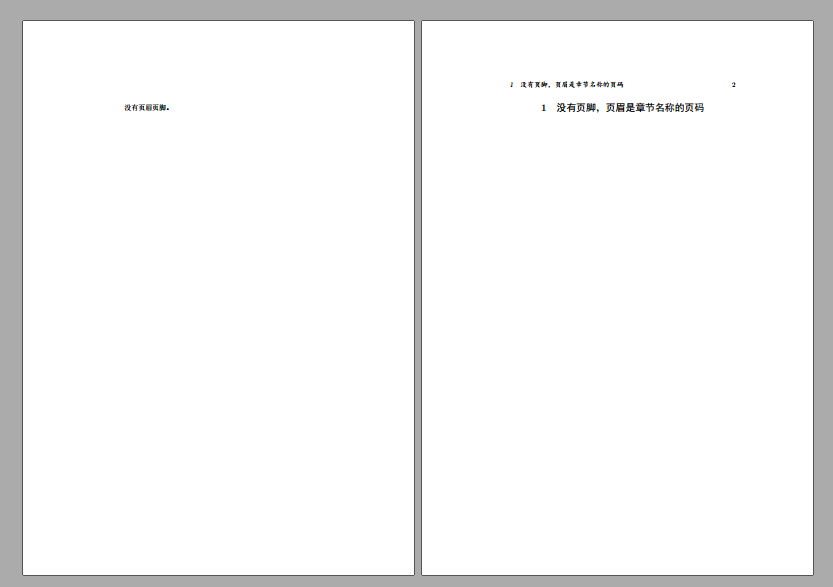$\qquad$ 另外，如想对页眉和页脚进行更多个性化的设置，可以去查阅宏包 $\mathtt{fancyhdr}$ 的使用。

# 目录链接

$\qquad$ 只需调用宏包 $\mathtt{hyperref}$ 即可。
$\qquad$ 但若是不对其加以设置，默认的样式是在有链接的文字上加红框，比较难看，所以下面简单解释这几个设置。
$\qquad$ 命令 $\mathbf{\backslash usepackage[option]\{hyperref\}}$ 提供的 $\mathtt{option}$ 主要有这两类：
$\qquad\qquad$ 1. $\mathtt{colorlinks}$ ，表示链接以改变字体颜色的形式而非加方框。
$\qquad\qquad$ 2. $\mathtt{linkcolor, anchorcolor, citecolor}$ ，分别表示用来标识 $\mathtt{link, anchor, cite}$ 等各种链接的颜色，对于目录的设置，我们使用的是 $\mathtt{linkcolor}$ 。
$\qquad$ 所以设置就显而易见了，若想使目录的链接颜色依旧是原本的黑色，则在 $\mathtt{option}$ 里设置 $\mathbf{[colorlinks,linkcolor=black]}$ 即可。
$\qquad$ 介于超链接不太好用图片表现，这里就不搞示例，可以直接去实践一下。
$\qquad$ 另外，该宏包还提供了几个生成超链接的命令，最常用的是这两个：
$\qquad\qquad$ 1. $\mathbf{\backslash url\{URL\}}$ ，生成网址链接，以等宽字体排版。
$\qquad\qquad$ 2. $\mathbf{\backslash href\{URL\}\{text\}}$ ，生成网址链接，以 $\mathtt{text}$ 里的内容显示描述，隐藏网址。
$\qquad$ 介于命令简单，这里不多加演示（暴露出懒的本性= =）。
$\qquad$ 另外，如想对目录进行更加深入的设置，可以去查阅宏包 $\mathtt{titletoc}$ 的使用。
$\qquad$ 既然讲到目录了就顺便说下，想对标题进行更多设置，可以去查阅宏包 $\mathtt{titlesec}$ 的使用。

# 行间距

$\qquad$ 调整行距可以使用宏包 $\mathtt{setspace}$ 。
$\qquad$ 定义环境 $\mathbf{\backslash begin\{spacing\}\{factor\}\ \backslash end\{spacing\}}$ ，在该环境内，所有行距都变为你设置的 $\mathtt{factor}$ 乘上默认行距， $\mathtt{factor}$ 是一个小数，不过貌似是有范围的（我用的时候感觉是有的），但因为我并没有在网上查阅到关于它的资料，就只能作罢。
$\qquad$ 而在我自己的测试中，在 $\mathtt{ctexaret}$ 模板下的默认行距实际上是介于 $1.1 \sim 1.2$ 之间的，不过我并没有找到相关资料，并不清楚这个数值的准确含义，因此我这里姑且当它是乘以默认行距的距离。
$\qquad$ 如有知道的麻烦在评论区回复或是私信我，谢谢。
$\qquad$ 示例代码：

\documentclass[UTF8]{ctexart}
\usepackage{setspace}
\begin{document}
%姑且当它是乘以默认行距的距离
\begin{spacing}{1.5}
这里是 $1.5$ 倍（相对默认的行距）行距这里是 $1.5$ 倍（相对默认的行距）行距这里是 $1.5$ 倍（相对默认的行距）行距这里是 $1.5$ 倍（相对默认的行距）行距\\
\end{spacing}
\begin{spacing}{0.5}
这里是 $0.5$ 倍（相对默认的行距）行距这里是 $0.5$ 倍（相对默认的行距）行距这里是 $0.5$ 倍（相对默认的行距）行距这里是 $0.5$ 倍（相对默认的行距）行距\\
\end{spacing}
\end{document}

$\qquad$ 效果预览：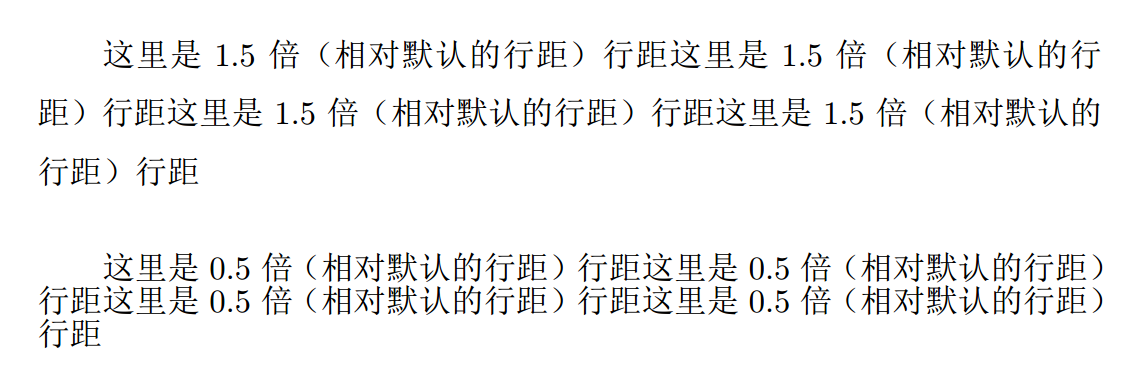$\qquad$ 另外，在导言区添加命令 $\mathbf{\backslash linespread\{factor\}}$ 是可以更改全文行距的，不过这个命令不晓得是为什么在编辑器里没有补全，且标注着命令错误的色块，但能过编译且是有效果的（该命令不是这里说的宏包里的）。
$\qquad$ 而设置行间距的命令不可能就这几个（这个宏包里就有挺多的），不过这里就不多讲了，有兴趣的可以去查阅资料。

# 文本位置

$\qquad$ 均是通过定义环境实现。
$\qquad$ 环境 $\mathbf{\backslash begin\{flushleft\}\ \backslash end\{flushleft\}}$ 会使其内的文本全部居左（左对齐）。
$\qquad$ 环境 $\mathbf{\backslash begin\{center\}\ \backslash end\{center\}}$ 会使其内的文本全部居中。
$\qquad$ 环境 $\mathbf{\backslash begin\{flushright\}\ \backslash end\{flushright\}}$ 会使其内的文本全部居右（右对齐）。
$\qquad$ 示例代码：

\documentclass[UTF8]{ctexart}
\begin{document}
\begin{flushleft}
\noindent 这里的文本是居左的（左对齐）。\\
这里的文本是居左的（左对齐）。\\
这里的文本是居左的（左对齐）。\\
\end{flushleft}
\begin{center}
这里的文本是居中的。\\
这里的文本是居中的。\\
这里的文本是居中的。\\
\end{center}
\begin{flushright}
这里的文本是居右的（右对齐）。\\
这里的文本是居右的（右对齐）。\\
这里的文本是居右的（右对齐）。\\
\end{flushright}
\end{document}

$\qquad$ 效果预览：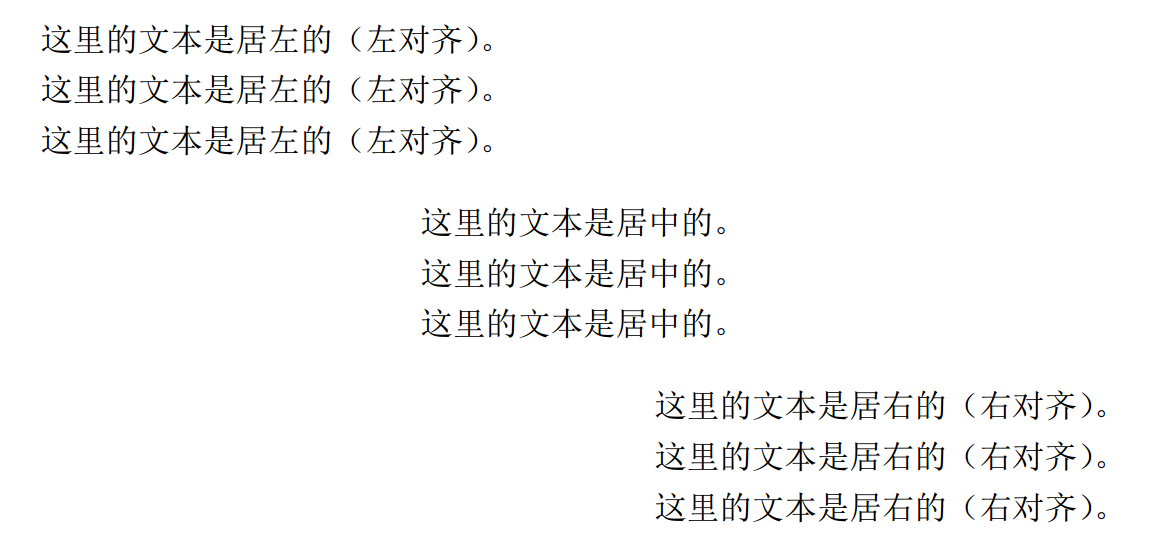# 给文本加线框

$\qquad$ 给文本加线框的方法有很多种，这里就简单介绍几种。
$\qquad$ $\mathbf{\backslash fbox\{text\}}$ ，该命令会给大括号里的文本 $\mathtt{text}$ 加上线框。
$\qquad$ 使用宏包 $\mathtt{framed}$ 。
$\qquad$ 环境 $\mathbf{\backslash begin\{framed\}\ \backslash end\{framed\}}$ 会给其内的文本加线框（建议配合居中环境使用）。
$\qquad$ 而有一个命令是专门给数学公式加框的，在我写的数学公式大全里有提到，这里就不写了。
$\qquad$ 示例代码：

\documentclass[UTF8]{ctexart}
\usepackage{framed}
\begin{document}
\fbox{这里使用 $\backslash$ fbox加线框}\\
\begin{center}
\begin{framed}
这里使用framed环境加线框。\\
\end{framed}
\end{center}
\end{document}

$\qquad$ 效果预览：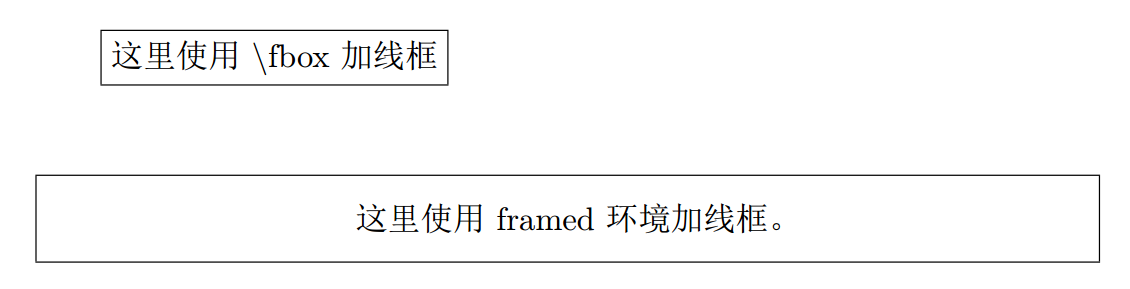$\qquad$ 其实 $\mathbf{\backslash fbox}$ 命令也可以和另外几个命令如 $\mathbf{\backslash parbox}$ 配合使用，但因为该命令比较“脆弱”，且不适合给较长的文本加框，所以这里就不介绍了。
$\qquad$ 另外，如想对线框进行更多个性化的设置，可以去查阅宏包 $\mathtt{mdframed}$ 的使用。

# 页面大小和页边距

$\qquad$ 关于设置页面大小在之前模板一栏已提到，这里不再阐述。
$\qquad$ 要设置页边距需调用宏包 $\mathtt{geometry}$ 。
$\qquad$ $\mathbf{\backslash geometry\{options\}}$ ，该命令可以自由设置页面上下左右的边距，在 $\mathtt{options}$ 中写的是 $\mathtt{left,right,top,bottom}$ ，分别是设置左、右、上、下边距。
$\qquad$ 例如 $\mathbf{\backslash geometry\{left=3.18cm,right=3.18cm,top=2.54cm,bottom=2.54cm\}}$ 就设置左右边距为 $\mathtt{3.18cm}$ ，上下边距为 $\mathtt{2.54cm}$ （其实这是 $\mathtt{word}$ 里默认的页边距）。
$\qquad$ 注意该命令是写在导言区的。

# 字体

$\qquad$ 介于我极少使用关于字体的命令，因此这里的介绍可能会有比较多的缺漏，请见谅。

## 英文字体

$\qquad$ 使用 $\mathtt{fontspec}$ 宏包。
$\qquad$ 常用的是下列 $3$ 个命令：
$\qquad\qquad$ 1. $\mathbf{\backslash setmainfont\{fontname\}}$ ，设置文章中西文部分默认使用的字体。
$\qquad\qquad$ 2. $\mathbf{\backslash setsansfont\{fontname\}}$ ，设置无衬线字体，可能出现在标题部分。
$\qquad\qquad$ 3. $\mathbf{\backslash setmonofont\{fontname\}}$ ，设置等宽字体。
$\qquad$ 这几个命令会将其后面对应文本的字体修改，若只想修改一部分文本的字体，只需用大括号将命令和要修改的文本括起来就好了。
$\qquad$ 另外，这几个命令都是调用的你本机的字体，所以用此命令也可直接调用本目录下的字体，如： $\mathbf{\backslash setmainfont\{[xxx.ttf]\}}$ 就调用了本目录下的 $\mathtt{xxx}$ 字体。
$\qquad$ 而这几个命令前可以进行设置（使用方括号）在对应的文本中若出现粗体、斜体之类的该使用什么字体，但该宏包通常能自动选择对应的字体，所以这里就不讲了，有需要的可以去查阅资料。
$\qquad$ 示例代码（因为一些原因就只演示第一个命令）：

\documentclass[UTF8]{ctexart}
\usepackage{fontspec}
\begin{document}
\noindent Default\\
\setmainfont{Times New Roman}
Times New Roman\\
\setmainfont{Courier}
Courier\\
\end{document}

$\qquad$ 效果预览：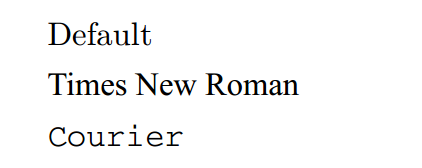## 中文字体

$\qquad$ 在模板为 $\mathtt{ctexart}$ 的时候是可以直接使用下列 $3$ 个修改中文字体的命令：
$\qquad\qquad$ 1. $\mathbf{\backslash setCJKmainfont\{fontname\}}$
$\qquad\qquad$ 2. $\mathbf{\backslash setCJKsansfont\{fontname\}}$
$\qquad\qquad$ 3. $\mathbf{\backslash setCJKmonofont\{fontname\}}$
$\qquad$ 这几个命令只能用于导言区的样子，且不晓得是为什么在编辑器里没有补全，并标注着命令错误的色块，但能过编译且是有效果的。
$\qquad$ 而由于中文字体一般是独立的，所以通常需要自己规定粗体时使用什么字体，如： $\mathbf{\backslash setCJKmainfont[BoldFont = \text{华文中宋}, ItalicFont = \text{华文楷体}]\{\text{华文宋体}\}}$ 表示默认使用华文宋体，粗体使用华文中宋，斜体使用华文楷体。
$\qquad$ 另外，实际上粗体、斜体等字体形状这些概念源于英文，中文并没有那么复杂，比如中文的斜体实际上都是单纯将字面从正方形改为平行四边形的“伪斜体”，因此在 $\mathtt{LaTeX}$ 里，常以楷体表示斜体（好像默认就是的）。若想使用伪斜体，请查阅相关的资料。
$\qquad$ 关于改变英文或中文字体的命令我了解的不多，因此对这两个条目更建议去查阅更多资料，我这里的介绍肯定是有很多缺漏的。

## 字体族

$\qquad$ $\mathtt{LaTeX}$ 默认提供了 $3$ 种字体族（针对英文）：
$\qquad\qquad$ 1. $\mathbf{\backslash textrm\{text\}}$ ，罗马（为默认）。
$\qquad\qquad$ 2. $\mathbf{\backslash textsf\{text\}}$ ，无衬线。
$\qquad\qquad$ 3. $\mathbf{\backslash texttt\{text\}}$ ，打字机。
$\qquad$ 示例代码：

\documentclass[UTF8]{ctexart}
\begin{document}
\noindent \textrm{Roman font family 罗马}\\
\textsf{Sans serif font family 无衬线}\\
\texttt{Typewriter font family 打字机}\\
\end{document}

$\qquad$ 效果预览：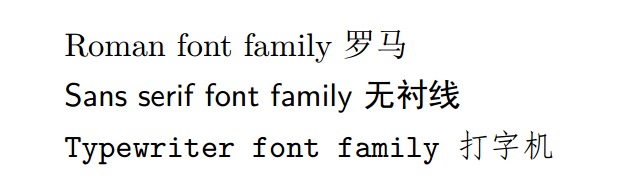## 字体形状

$\qquad$ $\mathtt{LaTeX}$ 默认提供了 $4$ 种字体形状：
$\qquad\qquad$ 1. $\mathbf{\backslash textup\{text\}}$ ，直立（为默认）。
$\qquad\qquad$ 2. $\mathbf{\backslash textit\{text\}}$ ，意大利体。
$\qquad\qquad$ 3. $\mathbf{\backslash textsl\{text\}}$ ，倾斜。
$\qquad\qquad$ 4. $\mathbf{\backslash textsc\{text\}}$ ，小型大写。
$\qquad$ 示例代码：

\documentclass[UTF8]{ctexart}
\begin{document}
\noindent \textup{Upright shape 直立}\\
\textit{Italic shape 意大利}\\
\textsl{Slanted shape 倾斜}\\
\textsc{Small capitals shape 小型大写}\\
\end{document}

$\qquad$ 效果预览：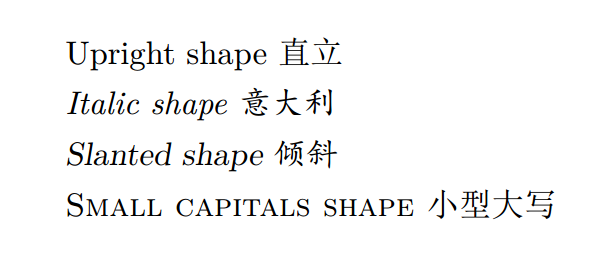## 字体系列

$\qquad$ $\mathtt{LaTeX}$ 默认提供了 $2$ 种字体系列：
$\qquad\qquad$ 1. $\mathbf{\backslash textmd\{text\}}$ ，中等权重（为默认）。
$\qquad\qquad$ 2. $\mathbf{\backslash textbf\{text\}}$ ，加粗。
$\qquad$ 示例代码：

\documentclass[UTF8]{ctexart}
\begin{document}
\noindent \textmd{Medium series 中等权重}\\
\textbf{Bold series 加粗}\\
\end{document}

$\qquad$ 效果预览：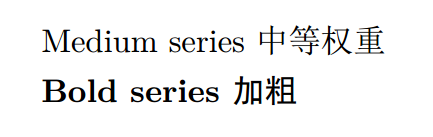## 字号

$\qquad$ 默认的字号大小是在模板前的设置里定义的，在之前有提到过，这里不多讲。
$\qquad$ 而 $\mathtt{LaTeX}$ 默认提供了 $10$ 种更改局部的文字大小的命令（按从小到大排列）：
$\qquad\qquad$ 1. $\mathbf{\backslash tiny}$
$\qquad\qquad$ 2. $\mathbf{\backslash scriptsize}$
$\qquad\qquad$ 3. $\mathbf{\backslash footnotesize}$
$\qquad\qquad$ 4. $\mathbf{\backslash small}$
$\qquad\qquad$ 5. $\mathbf{\backslash normalsize}$ ，为默认。
$\qquad\qquad$ 6. $\mathbf{\backslash large}$
$\qquad\qquad$ 7. $\mathbf{\backslash Large}$
$\qquad\qquad$ 8. $\mathbf{\backslash LARGE}$
$\qquad\qquad$ 9. $\mathbf{\backslash huge}$
$\qquad\qquad$ 10. $\mathbf{\backslash Huge}$
$\qquad$ 这几个命令会将其后面对应文本的字号修改，若只想修改一部分文本的字号，只需用大括号将命令和要修改的文本括起来就好了。
$\qquad$ 另外，这几个命令的大小是相对的，是以默认的字号为基准，而非固定的大小。
$\qquad$ 示例代码：

\documentclass[UTF8]{ctexart}
\begin{document}
\noindent {\tiny tiny 最小}\\
{\scriptsize scriptsize 脚本大小}\\
{\footnotesize footnotesize 脚注大小}\\
{\small small 小}\\
{\normalsize normalsize 正常大小}\\
{\large large 较大}\\
{\Large Large 大}\\
{\LARGE LARGE 很大}\\
{\huge huge 巨大}\\
{\Huge Huge 最大}\\
\end{document}

$\qquad$ 效果预览：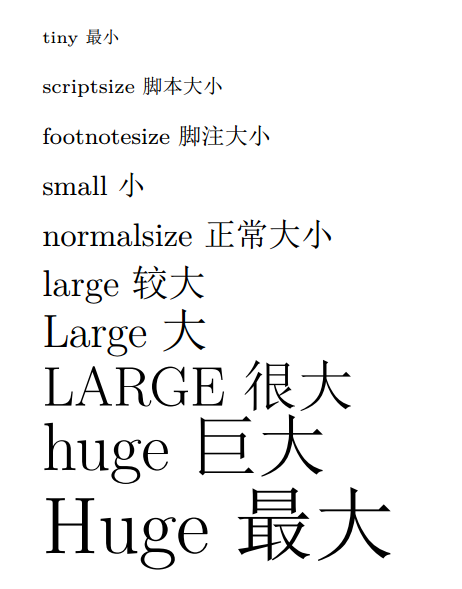## 划线

$\qquad$ 介于 $\mathtt{LaTeX}$ 提供的下划线命令不能自动换行，建议使用宏包 $\mathtt{ulem}$ ，其中还提供了一些划线的命令：
$\qquad\qquad$ 1. $\mathbf{\backslash uline\{text\}}$ ，下划线。
$\qquad\qquad$ 2. $\mathbf{\backslash uuline\{text\}}$ ，双下划线。
$\qquad\qquad$ 3. $\mathbf{\backslash uwave\{text\}}$ ，波浪线。
$\qquad\qquad$ 4. $\mathbf{\backslash sout\{text\}}$ ，删除线。
$\qquad\qquad$ 5. $\mathbf{\backslash xout\{text\}}$ ，斜删除线。
$\qquad\qquad$ 6. $\mathbf{\backslash dashuline\{text\}}$ ，虚线。
$\qquad\qquad$ 7. $\mathbf{\backslash dotuline\{text\}}$ ，点线。
$\qquad$ 示例代码：

\documentclass[UTF8]{ctexart}
\usepackage{ulem}
\begin{document}
\noindent \uline{uline 下划线}\\
\uuline{uuline 双下划线}\\
\uwave{uwave 波浪线}\\
\sout{sout 删除线}\\
\xout{xout 斜删除线}\\
\dashuline{dashuline 虚线}\\
\dotuline{dotuline 点线}\\
\end{document}

$\qquad$ 效果预览：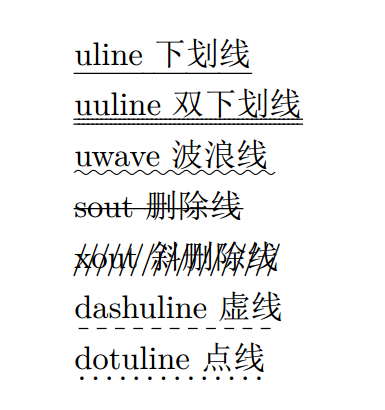$\qquad$ 另外，该宏包还修改了部分 $\mathtt{LaTeX}$ 默认的命令的效果，若想使用原来的效果，可以在调用宏包时加上设置 $\mathtt{normalem}$ 即可。

# 插入代码

$\qquad$ 建议使用宏包 $\mathtt{listings}$ 。
$\qquad$ 环境 $\mathbf{\backslash begin\{lstlisting\}\ \backslash end\{lstlisting\}}$ 内填写代码。
$\qquad$ 在 $\mathbf{\backslash lstset\{\}}$ 里填写对代码块的设置，该命令用于导言区，是对代码块的全局设置，通常我们都会配好一个设置，而非对每个代码块去局部设置，所以这里就不讲局部设置了。
$\qquad$ 而关于代码块的设置，常用的命令可以参考下面的示例代码。
$\qquad$ 注意要设置代码块中文字和背景的颜色时，建议引入宏包 $\mathtt{xcolor}$ 来设置，这里对该宏包暂不介绍，反正一般直接用里面的 $\mathtt{RGB}$ 进行设置，有需要的可以去网上查阅。
$\qquad$ 要设置代码块中文字字体，使用上文提到的 $\mathtt{fontspec}$ 包即可。
$\qquad$ 示例代码：

\documentclass[UTF8]{ctexart}
\usepackage{listings}
\usepackage{xcolor}
\usepackage{fontspec}
\lstset{
basicstyle={                                % 设置代码格式
\fontspec{Consolas}
\scriptsize
},
keywordstyle={                              % 设置关键字格式
\color[RGB]{40,40,255}
\fontspec{Consolas Bold}
\scriptsize
},
stringstyle={                               % 设置字符串格式
\color[RGB]{128,0,0}
\fontspec{Consolas}
\scriptsize
},
\color[RGB]{0,96,96}
\fontspec{Consolas}
\scriptsize
},
numberstyle={                               % 设置行号格式
\scriptsize
\color{black}
\fontspec{Consolas}
},
emphstyle=\color[RGB]{112,64,160},          % 设置强调字格式
language=c++,                               % 设置语言
numbers=left,                               % 显示行号
%   numbersep=5pt,                              % 设置行号与代码的距离
frame=single,                               % 设置背景边框
tabsize=4,                                  % 设置tab长度
backgroundcolor=\color[RGB]{245,245,244},   % 设定背景颜色
showstringspaces=false,                     % 不显示字符串中的空格
showspaces=false,                           % 不显示代码中的空格
showtabs=false,                             % 不显示代码中的tab
breaklines=true,                            % 设置自动换行
morekeywords={},                            % 可以手动添加关键字
emph={scanf,printf},                        % 可以手动添加强调字
%   xleftmargin=2em,                            % 设置左边距，默认与页芯等宽
%   xrightmargin=2em,                           % 设置右边距，默认与页芯等宽
%   aboveskip=1em,                              % 设置上边距
}
\begin{document}
\begin{lstlisting}
#include<cstdio>
using namespace std;
int main()
{
int x, y;
scanf("%d%d", &x, &y);
printf("%d", x + y);
return 0;
}
\end{lstlisting}
\end{document}

$\qquad$ 效果预览：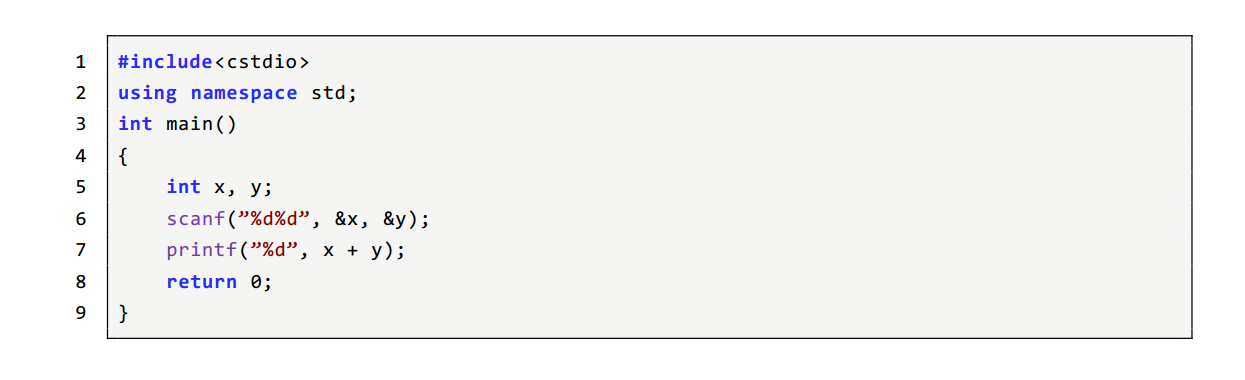$\qquad$ 关于对代码块更多的设置，可以去查阅该宏包的文档。

# 较复杂表格

## 基本设置

$\qquad$ 先看基本命令：
$\qquad\qquad$ $\mathbf{\backslash begin\{{tabular\}[pos]\{cols\}}\ \backslash end\{tabular\}}$
$\qquad$ $\mathtt{cols}$ 里填写的内容在先前有简单解释，现在再简单归纳并讲下这几个常用的：
$\qquad\qquad$ 1. $\mathtt{l}$ ，该列单元为左对齐。
$\qquad\qquad$ 2. $\mathtt{c}$ ，该列单元为居中。
$\qquad\qquad$ 3. $\mathtt{r}$ ，该列单元为右对齐。
$\qquad\qquad$ 4. $\mathtt{p\{wide\}}$ ，固定该列宽度，且若单元的内容过长会自动换行，实际上称为段落列，文本在顶部对齐，另外还有两种不同对齐方式的段落列，但需要引入宏包 $\mathtt{array}$ ，这里暂不展开。
$\qquad\qquad$ 5. $\mathtt{|}$ ，画一条竖线。
$\qquad$ 示例代码：

\documentclass[UTF8]{ctexart}
\begin{document}
\begin{center}
\begin{tabular}{|l|c|r|p{5cm}|}
\hline
左对齐 & 居中 & 右对齐 & 固定列宽 \\
\hline
l & c & r & p \\
\hline
\end{tabular}
\end{center}
\end{document}

$\qquad$ 效果预览：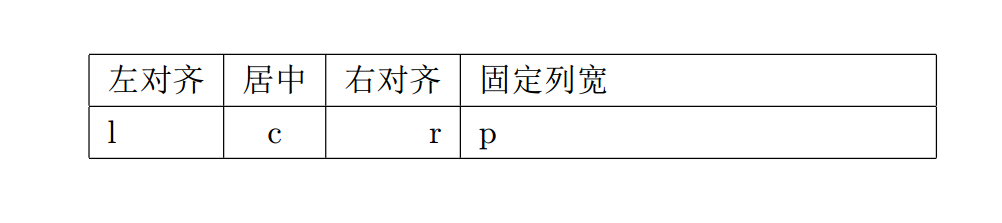$\qquad$ $\mathtt{pos}$ 选项通常用于设置表格相对于周围文本基线的纵向位置，但一般不使用这个选项，这里就不介绍了。
$\qquad$ 环境中的基本命令在先前也有简单解释，现在再简单归纳并讲下这几个常用的：
$\qquad\qquad$ 1. $\&$ ，分隔列。
$\qquad\qquad$ 2. $\backslash\backslash$ ，分隔行。
$\qquad\qquad$ 3. $\mathbf{\backslash hline}$ ，画一条水平线。
$\qquad\qquad$ 4. $\mathbf{\backslash newline}$ ，表格单元内换行，只能在段落列里使用。
$\qquad\qquad$ 5. $\mathbf{\backslash cline\{i-j\}}$ ，从第 $i$ 列到第 $j$ 列画一条水平线，一般配合单元格合并使用，因为将在下文讲，这里就不演示了。
$\qquad$ 示例代码：

\documentclass[UTF8]{ctexart}
\begin{document}
\begin{center}
\begin{tabular}{|p{3cm}|p{5cm}|}
\hline
先前忘记演示自动换行了 & 在表格单元里 \newline 换行 \\
\hline
在这里补上好了 & newline \\
\hline
\end{tabular}
\end{center}
\end{document}

$\qquad$ 效果预览：$\qquad$ 因为 $\mathtt{LaTeX}$ 的格式化表格有一些缺陷，其中一个就是当单元内文字过长（甚至超过页面宽度），它不会自动换行，通常使用段落列来避免，若想使用更方便的方法，可以去查阅宏包 $\mathtt{tabularx}$ 或 $\mathtt{tabulary}$ 的使用。
$\qquad$ 若想为整列指定字体形状，可以去查阅宏包 $\mathtt{array}$ 的使用。
$\qquad$ 关于表格的行与行之间的距离，可以使用先前提到的宏包 $\mathtt{setspace}$ 进行粗略的调整，若想要更加精细的调整，可以去查阅相关资料。
$\qquad$ 而列之间的距离可以使用命令 $\mathbf{\backslash setlength\{\backslash tabcolsep\}\{length\}}$ 改变列距为 $\mathtt{length}$ （默认为 $\mathtt{5pt}$ ），该命令写于表格环境前。另外也可以使用命令 $\mathbf{\backslash hspace}$ 来控制，这里暂不介绍如何用它控制，有需要的可以去查阅资料。

## 单元格合并

$\qquad$ 先讲合并列，即一个单元格跨越多列。
$\qquad$ $\mathbf{\backslash multicolumn\{cols\}\{pos\}\{text\}}$
$\qquad$ $\mathtt{cols}$ 内填写你想要合并的列的数量（从该命令所处列往后数，包括该列）。
$\qquad$ $\mathtt{pos}$ 内填写的是和定义表格的命令的 $\mathtt{cols}$ 设置一样的东西，效果也是相同的。
$\qquad$ $\mathtt{text}$ 内填写文本。
$\qquad$ 示例代码：

\documentclass[UTF8]{ctexart}
\begin{document}
\begin{center}
\begin{tabular}{|c|c|c|}
\hline
\multicolumn{3}{|c|}{一个单元格跨越多列} \\
\hline
gu & \multicolumn{2}{|l}{左对齐且右侧无竖线} \\
\hline
\end{tabular}
\end{center}
\end{document}

$\qquad$ 效果预览：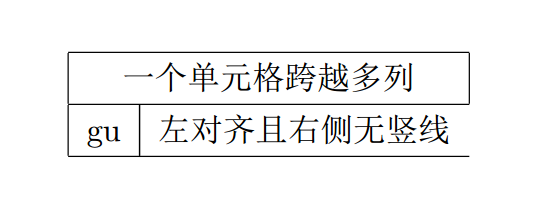$\qquad$ 合并行，即一个单元格跨越多行。
$\qquad$ 需要引入宏包 $\mathtt{multirow}$ 。
$\qquad$ $\mathbf{\backslash multirow\{number of rows\}\{width\}\{text\}}$
$\qquad$ $\mathtt{number of rows}$ 内填写你想要合并的行的数量（从该命令所处行往后数，包括该行）。
$\qquad$ $\mathtt{width}$ 内填写合并后单元格的宽度，若想其随文本长度变化而变化，请打上 $\ast$ 号。
$\qquad$ $\mathtt{text}$ 内填写文本。
$\qquad$ 需要注意的是，合并行的单元格在其跨越的后续单元格原来的位置必须留一个空位，详情可见示例代码。
$\qquad$ 示例代码（同时演示了 $\mathbf{\backslash cline\{i-j\}}$ 的使用）：

\documentclass[UTF8]{ctexart}
\usepackage{multirow}
\begin{document}
\begin{center}
\begin{tabular}{|c|c|c|}
\hline
\multirow{2}{100pt}{强制宽度} & 注意跨越的单元格要留空 & \multirow{3}{*}{合并行，宽度随文本变化}\\
\cline{2-2}
& 注意跨越的单元格要留空 &  \\
\cline{1-2}
gu & 注意跨越的单元格要留空 & \\
\hline
\end{tabular}
\end{center}
\end{document}

$\qquad$ 效果预览：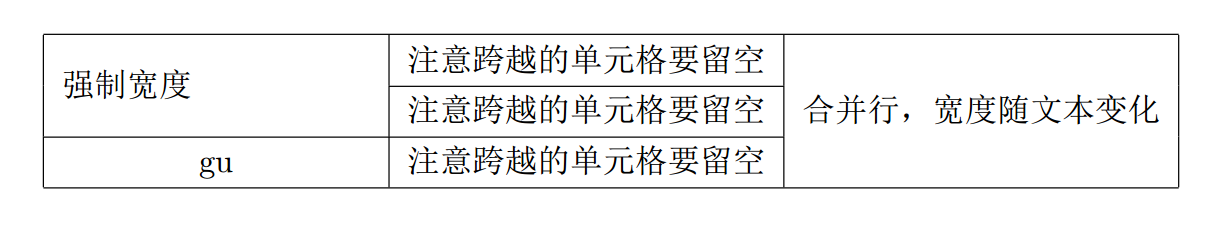$\qquad$ 至于要实现同时跨越行列，将两个命令结合起来即可，一些注意事项见示例代码。
$\qquad$ 示例代码：

\documentclass[UTF8]{ctexart}
\usepackage{multirow}
\begin{document}
\begin{center}
\begin{tabular}{|c|c|c|}
\hline
\multicolumn{2}{|c|}{\multirow{2}{*}{同时跨越行与列}} & 注意在跨越行的地方要使用合并列命令\\
\cline{3-3}
\multicolumn{2}{|c|}{} & 内容填为空 \\
\hline
并以该命令提供的 & pos设置来画竖线 & 建议自己实践下 \\
\hline
\end{tabular}
\end{center}
\end{document}

$\qquad$ 效果预览：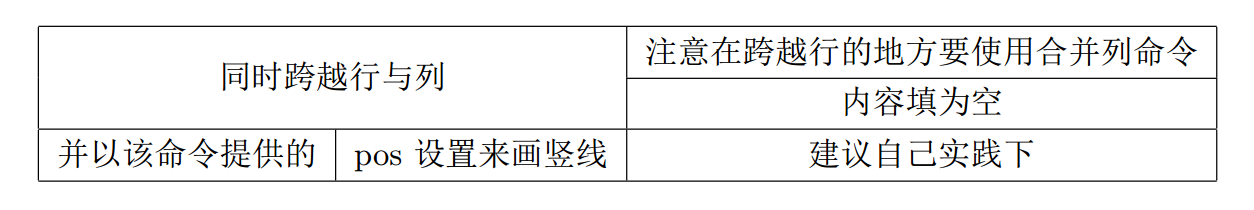$\qquad$ 另外，在 $\mathtt{TeXstudio}$ 菜单栏的向导一栏里，有表格向导，相当于一个图形化的制作表格页面，它会按你的要求自动插入对应的代码，颇为方便。
$\qquad$ 而该编辑器中也有快捷的对行列操作（默认位于菜单栏的图形按钮一栏）。
$\qquad$ 关于表格的更多设置，如单元格背景色等，可以去查阅资料，这里就不讲了。

# 浮动体

$\qquad$ 在实际撰写文稿的过程中，我们可能会碰到一些占据篇幅较大，但同时又不方便分页的内容，如图片和表格，通常就属于这样的类型。若不想直接插入导致溢出页面或是大片的空白的话，此时就需要使用浮动体来避免以上情况出现。
$\qquad$ 浮动体并不是普通文本流的一部分，而是一个单独分离出来的实体。浮动体通常都有描述它们的标题，以及编号，因此可以在文章的其它地方引用它们。
$\qquad$ 在 $\mathtt{LaTeX}$ 中，默认的浮动体有 $\mathtt{figure}$ 和 $\mathtt{table}$ 两种，另外也可以自己自定义一个浮动体。
$\qquad$ 这里仅以 $\mathtt{figure}$ 为例， $\mathtt{table}$ 的操作与其差不太多，至于自定义浮动体，通常并不需要用到，有需要的可以去查阅资料。

## 基本命令

$\qquad$ $\mathtt{figure}$ 浮动体定义在环境 $\mathbf{\backslash begin\{{figure\}[placement]}\ \backslash end\{figure\}}$ 中。
$\qquad$ $\mathtt{placement}$ 里填写的是该浮动体的位置描述符，即定义将该浮动体置于哪里，常用的有以下参数：
$\qquad\qquad$ 1. $\mathtt{h}$ ，即 $\mathtt{here}$ ，该浮动体会大致出现在原文本中的原来的位置（并非完全是）。
$\qquad\qquad$ 2. $\mathtt{t}$ ，即 $\mathtt{top}$ ，该浮动体会被尝试放在一页的顶部。
$\qquad\qquad$ 3. $\mathtt{b}$ ，即 $\mathtt{bottom}$ ，该浮动体会被尝试放在一页的底部。
$\qquad\qquad$ 4. $\mathtt{p}$ ，即 $\mathtt{float\ page}$ ，该浮动体会被尝试单独成页。
$\qquad$ 另外，若想禁止浮动体的浮动效果，可以使用宏包 $\mathtt{float}$ 提供的 $\mathtt{H}$ 选项。
$\qquad$ 需要注意的是，这些位置描述符只是你希望 $\mathtt{LaTeX}$ 这么做，而 $\mathtt{LaTeX}$ 会根据一种算法来找到它认为最适合的位置来放置浮动体，也就是说，在某些特殊情况下， $\mathtt{LaTeX}$ 并不会遵循这些位置描述符。

## 基本内容

$\qquad$ 在 $\mathtt{figure}$ 环境中填写的内容首先便是插入图片，依旧使用前文提到的 $\mathbf{\backslash includegraphics[\ ]\{\}}$ 命令即可。
$\qquad$ 其次就是添加标题，使用命令 $\mathbf{\backslash caption\{text\}}$ 添加标题， $\mathtt{text}$ 里即是标题内容，而该命令与插入图片的命令的相对位置决定了标题位于图片上方还是下方，具体可见示例代码。
$\qquad$ 另外，常使用 $\mathbf{\backslash centering}$ 使图表居中排版。
$\qquad$ 使用 $\mathbf{\backslash reflectbox\{text\}}$ 可以水平翻转里面的内容，包括图片。
$\qquad$ 示例代码：

\documentclass[UTF8]{ctexart}
\usepackage{graphicx}
\begin{document}
\begin{figure}[h]
\centering
\caption{标题在图片上方}
\includegraphics[width=5cm,height=4cm]{1.png}
\end{figure}
\begin{figure}[h]
\centering
\reflectbox{\includegraphics[width=5cm,height=4cm]{1.png}}
\caption{标题在图片下方，且图片被水平翻转}
\end{figure}
\end{document}

$\qquad$ 效果预览：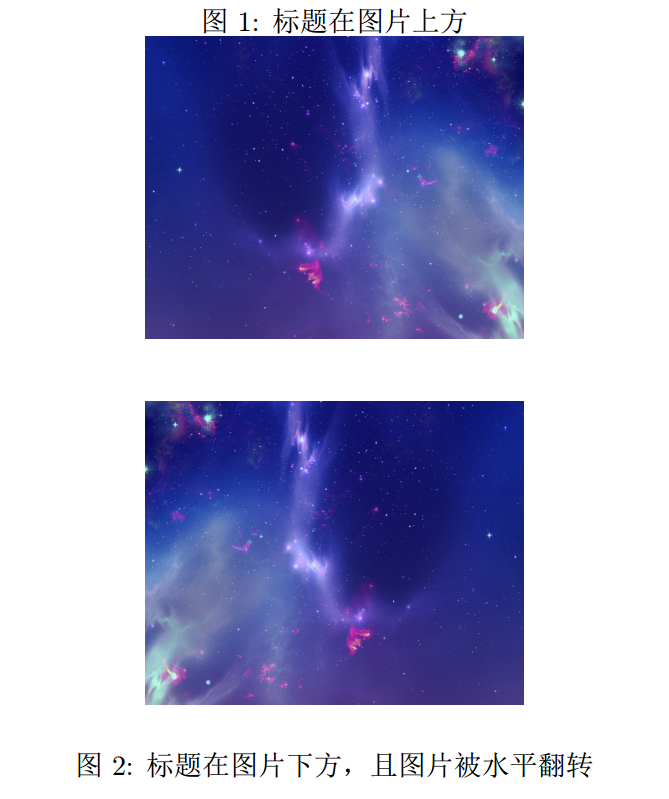$\qquad$ 如希望让标题在图表的侧面出现，可以去查阅宏包 $\mathtt{sidecap }$ 的使用。

## 图表的列表

$\qquad$ 使用 $\mathbf{\backslash listoffigures}$ 或 $\mathbf{\backslash listoftables}$ 可以在文章开头列出图表，通常会显示图表的标题、编号，以及它们所在的页码。
$\qquad$ 若是图表的标题是在过长，可以使用 $\mathbf{\backslash caption[short\ text]\{text\}}$ 提供的 $\mathtt{short\ text}$ ，在文章内容中实际显示的标题为后面的 $\mathtt{text}$ ，而在列表中显示 $\mathtt{short\ text}$ 。
$\qquad$ 示例代码：

\documentclass[UTF8]{ctexart}
\usepackage{graphicx}
\begin{document}
\listoffigures
\begin{figure}[h]
\centering
\includegraphics[width=5cm,height=4cm]{2.png}
\caption[这是一张图]{这是一张图片这是一张图片这是一张图片这是一张图片}
\end{figure}
\end{document}

$\qquad$ 效果预览：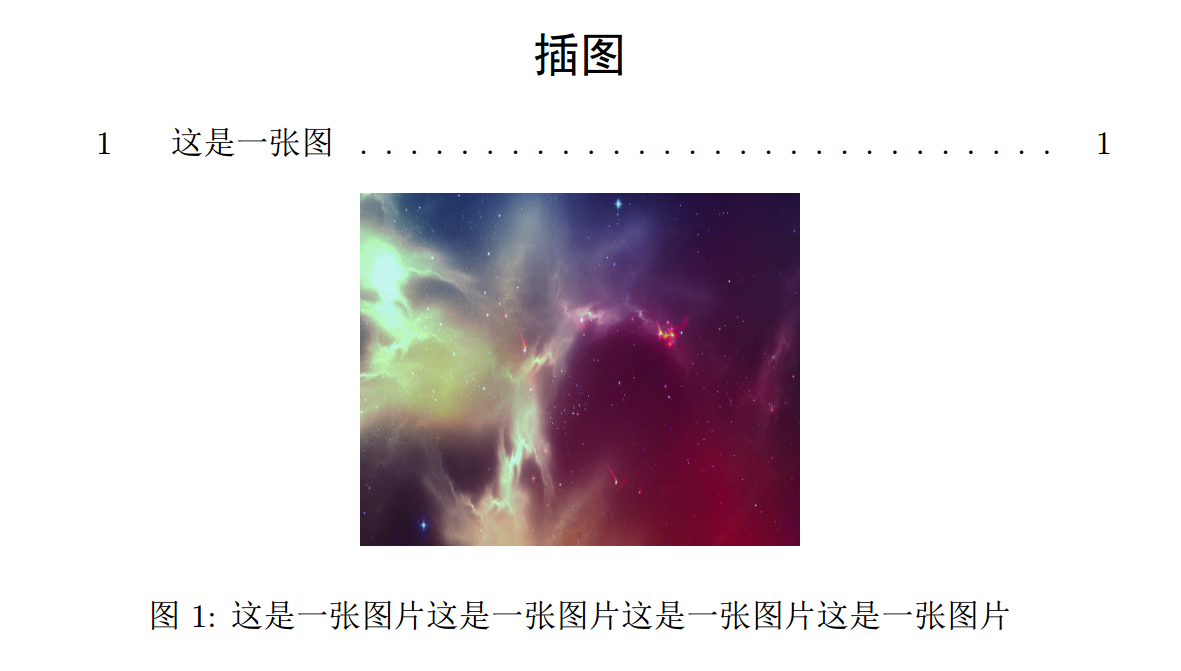$\qquad$ 另外，若希望浮动体能被文字所环绕而非独立，可以去查阅宏包 $\mathtt{wrapfig}$ 的使用。若想在浮动体中套子浮动体，可以去查阅宏包 $\mathtt{subcaption}$ 的使用。
$\qquad$ 另外，在 $\mathtt{TeXstudio}$ 菜单栏的向导一栏里，有插入图片的向导，相当于一个图形化的插入图片页面，它会按你的要求自动插入对应的浮动体或是普通图片，也是很方便的。

# 推荐网站

$\qquad$ $\mathtt{Wikibooks}$ 的 $\mathtt{LaTeX}$ 帮助书，很全面，内容多，不过是英文，看起来比较累。
$\qquad$ $\mathtt{TeXdoc\ Online}$ ，一个包含大部分宏包文档的在线查询网站，不过实际上在 $\mathtt{TeXstudio}$ 里，按住 $\mathtt{Ctrl}$ 再点击引用的宏包，就可以看到该宏包的文档了。
$\qquad$ $\mathtt{LaTeX}$ 工作站，一个中文网站，虽然文章良莠不齐，且大多是转载，但好歹算是中文资料的一个集合地，毕竟 $\mathtt{LaTeX}$ 的中文资料实在太少了。
$\qquad$ $\mathtt{LaTeX\ Stack\ Exchange}$ ，一个外国的关于 $\mathtt{LaTeX}$ 的交流网站。
$\qquad$ $\mathtt{Liam\ Huang}$ 大佬的博客，这个大佬的博客里有许多高质量的关于 $\mathtt{LaTeX}$ 的文章。
$\qquad$ 还有就是谷歌或百度，学习 $\mathtt{LaTeX}$ 最重要的就是多查阅资料。

# 参考资料

$\qquad$ $\mathtt{Wikibooks}$ 的 $\mathtt{LaTeX}$ 帮助书
$\qquad$ 一些宏包的文档和大量的博客，介于数量实在太多，这里不一一列举，若有需要可以私信我。
$\qquad$ 感谢所有参考资料给予我的帮助。

# 后记

$\qquad$ 其实呢，在开始写这篇文章的时候，我的初衷是写一篇简洁的入门就好，但写着写着，一边感叹 $\mathtt{LaTeX}$ 的博大精深，一边就发现我想写的内容越来越多，一发不可收拾，于是最后就写了那么多。
$\qquad$ 而你们在学习过程中很可能会遇到问题，但无论如何，都先去找相关资料阅读，如果依旧无法解决，可以去搜索软件搜索问题，再不行就去 $\mathtt{LaTeX}$ 的社区里询问。
$\qquad$ 关于我建议使用的编辑器 $\mathtt{TeXstudio}$ ，实际上有许多很好用很强大的功能，在这篇文章里我只是提了几个，更多的功能就要靠你们自己去发现了（当然也可以去查阅资料）。
$\qquad$ 总之，感谢你能读到此处，希望这篇文章能对你有所帮助。

$\qquad$ 如需转载，请注明原址。